In :
%matplotlib inline
import numpy as np
import pandas as pd
import statsmodels as sm
import seaborn as sns
import matplotlib.pyplot as plt

sns.set_context('notebook')

In :
np.random.seed(42)


Data import

In :
ts_data = pd.read_excel('Trend study data_DEC2012-2015.xlsx', index_col=0)

Out:
Percent_incident Outbreaks Moving AGE Case Average Moving Outbreak Average
Year_week
2012- 49 51.7241 1 25.86205 0.5
2012- 50 45.0000 0 48.36205 0.5
2012- 51 51.8519 1 48.42595 0.5
2012- 52 31.2500 0 41.55095 0.5
2012- 53 0.0000 0 15.62500 0.0

Plot of incidence time series (blue line) and the weekly outbreak records (green dots).

In :
ts_data.Percent_incident.plot(label='incidence')
ax = ts_data.Outbreaks.plot(secondary_y=True, style='o', label='outbreaks')
ax.set_yticks(range(3))
ax.set_ylim(0, 2.5);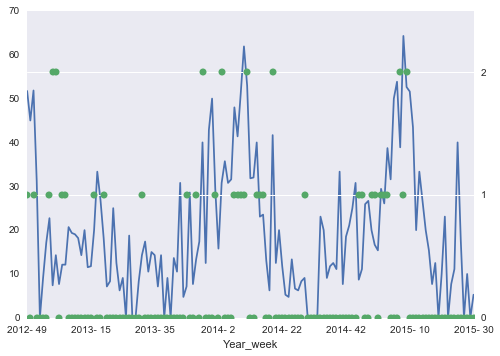Autocorrelation function and partial autocorrelation function for observed incidence. The partial autocorrelation shows the autocorrelation at a particular time lag, controlling for the autocorrelation of other periods.

This shows that there is probably information going back 2 or 3 weeks that can help us inform the prediction of incidence.

In :
from statsmodels.graphics.tsaplots import acf, pacf

fig, axes = plt.subplots(1, 2)
a = acf(ts_data.Percent_incident)
axes.bar(range(len(a)), a)
axes.set_ylim(-1,1)
axes.set_title('ACF')

p = pacf(ts_data.Percent_incident)
axes.bar(range(len(p)), p)
axes.set_ylim(-1,1)
axes.set_title('Partial ACF')

Out:
<matplotlib.text.Text at 0x10981d860>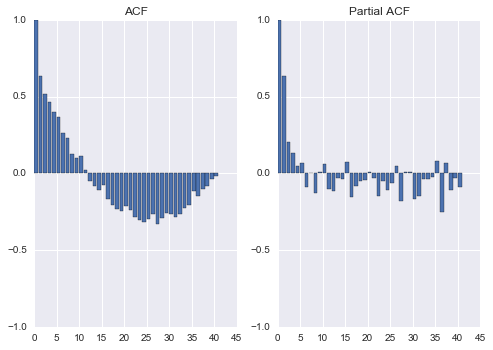This model fits a second order autoregressive model to the incidence time series to check how well current incidence can be predicted from the previous two weeks. That is, we fit the following relationship:

$$\theta_t = \mu + \rho_1 y_{t-1} + \rho_2 y_{t-2}$$

where the $\rho_1, \rho_2$ parameters are the influence of information one week and two weeks prior, respectively, on the current expected incidence ($\theta_t$).

We then use this value in a normal likelihood to predict the observed value $y_t$:

$$y_t \sim N(\theta_t, \tau$$

In :
import pymc3 as pm

y = ts_data.Percent_incident.values

with pm.Model() as ar2:

mu = pm.Normal('mu', 0, 1e-6)

# Autoregressive parameters
ar = 2
rho = pm.Normal('rho', 0, 1e-3, shape=ar)

sigma = pm.Uniform('sigma', 0, 100)
tau = sigma ** -2

# Expected values
theta = pm.Deterministic('theta', mu + rho*y[:-2] + rho*y[1:-1])

incid = pm.Normal('incid', theta, tau, observed=y[ar:])
incid_pred = pm.Normal('incid_pred', theta, tau, shape=y[ar:].shape)

In :
with ar2:

tr = pm.sample(2000)

Assigned <class 'pymc3.step_methods.nuts.NUTS'> to mu
Assigned <class 'pymc3.step_methods.nuts.NUTS'> to rho
Assigned <class 'pymc3.step_methods.nuts.NUTS'> to sigma_interval
Assigned <class 'pymc3.step_methods.nuts.NUTS'> to incid_pred
[-----------------100%-----------------] 2000 of 2000 complete in 3.5 sec
In :
pm.traceplot(tr[1000:], vars=['rho', 'sigma']);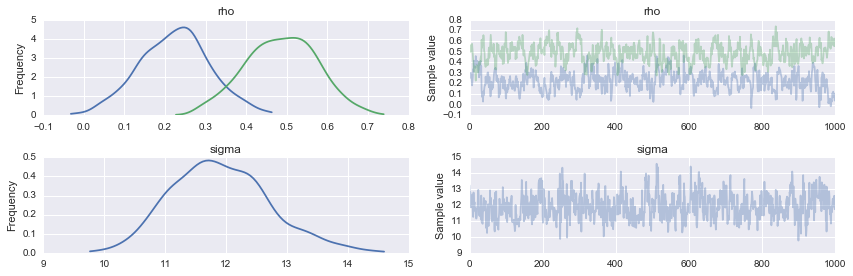Expected incidence

In :
ax = ts_data.Percent_incident.plot()
ax.plot(tr[-100:]['theta'].T, alpha=0.02, color='r');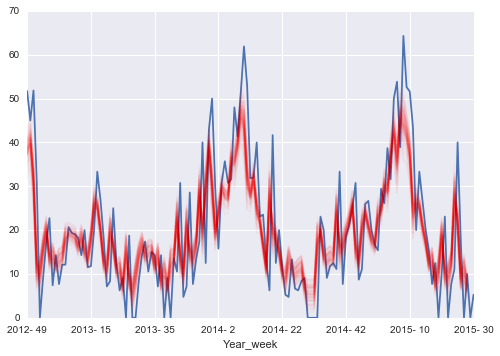Predicted incidence, that is, including sampling variation as well as parametric uncertainty.

In :
ax = ts_data.Percent_incident.plot()
ax.plot(tr[-100:]['incid_pred'].T, alpha=0.02, color='r');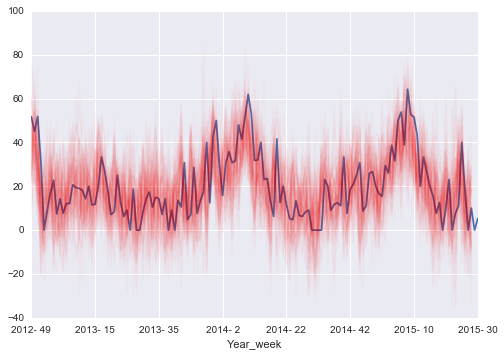Now that we have shown that we can predict current incidence from past incidence, we extend the model by attempting to predict outbreaks based on the predicted incidence.

Specifically, we model the observed number of outbreaks in week $t$ as a negative binomial random variable. The negative binomial is a generalization of the Poisson distribution that can be used to flexibly predict counts.

$$x_t \sim NB(\phi_t, \alpha)$$

where the expected value $\phi_t$ is a function of the expected incidence that we predicted from past incidence.

$$\phi_t = \beta_0 + \beta_1 \theta_t$$

For this model, the prediction is based on the previous three weeks of incidence, rather than just 2 in the previous model.

In :
import pymc3 as pm
import theano.tensor as tt

y = ts_data.Percent_incident.values
count = ts_data.Outbreaks

with pm.Model() as ar2_pred:

mu = pm.Normal('mu', 0, 1e-6)

# Autoregressive parameters
ar = 3
rho = pm.Normal('rho', 0, 1e-3, shape=ar)

# Expected values
theta = pm.Deterministic('theta', mu + rho*y[:-ar] + rho*y[ar-2:-(ar-1)]
+ rho*y[ar-1:-(ar-2)])

sigma = pm.Uniform('sigma', 0, 100)
tau = sigma ** -2

incid = pm.Normal('incid', theta, tau, observed=y[ar:])

beta = pm.Normal('beta', 0, 0.01, shape=2)

expected_outbreaks = pm.Deterministic('expected_outbreaks', np.exp(beta + beta*theta))
# Probability of one or more outbreaks
pred_prob_outbreak = pm.Deterministic('pred_prob_outbreak', expected_outbreaks>1)

alpha = pm.Exponential('alpha', 0.1, testval=1)

outbreaks = pm.NegativeBinomial('outbreaks', expected_outbreaks, alpha=alpha,
observed=count[ar:])

In :
iterations = 10000
burn = 5000

In :
with ar2_pred:
start = pm.find_MAP()
trace = pm.sample(iterations, step=pm.NUTS(scaling=start), start=start)

 [-----------------100%-----------------] 10000 of 10000 complete in 83.4 sec
In :
pm.traceplot(trace[burn:], vars=['beta', 'alpha']);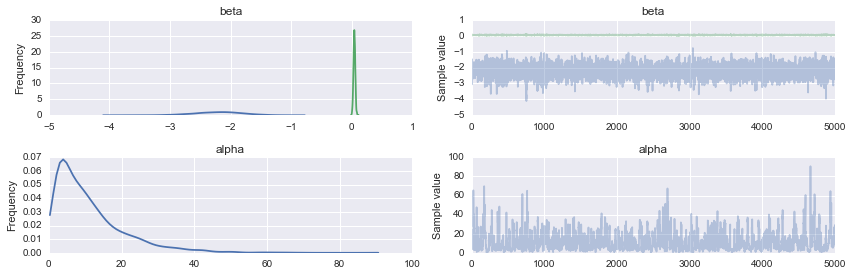In :
pm.summary(trace[burn:], vars=['rho', 'beta'])

rho:

Mean             SD               MC Error         95% HPD interval
-------------------------------------------------------------------

0.128            0.084            0.001            [-0.038, 0.288]
0.157            0.093            0.001            [-0.033, 0.334]
0.444            0.086            0.001            [0.269, 0.604]

Posterior quantiles:
2.5            25             50             75             97.5
|--------------|==============|==============|--------------|

-0.037         0.074          0.128          0.183          0.291
-0.028         0.095          0.158          0.219          0.343
0.274          0.387          0.445          0.503          0.610

beta:

Mean             SD               MC Error         95% HPD interval
-------------------------------------------------------------------

-2.206           0.402            0.006            [-2.981, -1.423]
0.044            0.015            0.000            [0.014, 0.073]

Posterior quantiles:
2.5            25             50             75             97.5
|--------------|==============|==============|--------------|

-3.025         -2.468         -2.191         -1.931         -1.455
0.014          0.034          0.044          0.054          0.074


In :
ax = ts_data.Outbreaks.plot(style='bo')
ax.plot(trace[-100:]['expected_outbreaks'].T, alpha=0.02, color='r');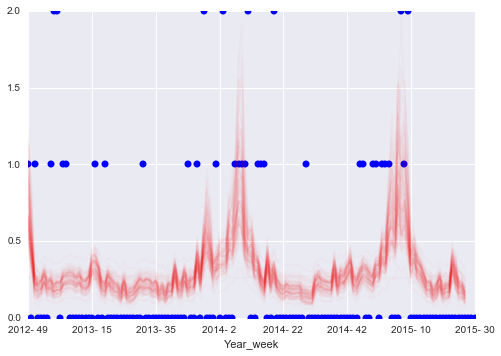This plots the posterior probability of 1 or more outbreaks, based on incidence information in the previous 3 weeks. The labels indicate the year and week of times where the probability of 1 or more outbreaks is predicted to be greater than 5%.

In :
probs = trace[burn:]['pred_prob_outbreak'].mean(0)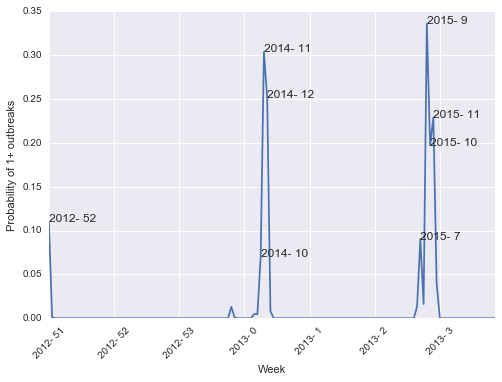!gist -u 670e777406a2f2bfb67e "Norovirus prediction.ipynb"